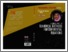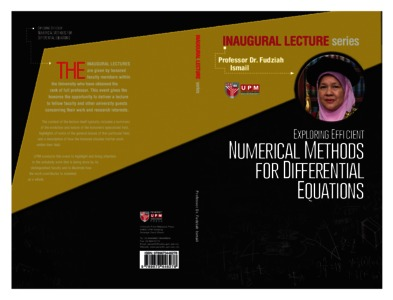# Exploring efficient: numerical methods for differential equations

## Citation

Ismail, Fudziah (2016) Exploring efficient: numerical methods for differential equations. [Inaugural Lecture]

## Abstract

Numerical analysis is a way to do higher mathematical problems on a computer, a technique widely used by scientists and engineers to solve their problems. A major advantage of numerical analysis is that a numerical answer can be obtained even when a problem has no “analytical” solution. Results from numerical analysis are an approximation, which can be made as accurate as desired. The analysis of errors in numerical methods is a critically important part of the study of numerical analysis. Hence, we will see in this research that computation of the error is a must as it is a way to measure the efficiency of the numerical methods developed. Numerical methods require highly tedious and repetitive computations that can only be done using the computer. Hence in this research, it is shown that computer programs must be written for the implementation of numerical methods. In the early part of related research the computer language used was Fortran. Subsequently more and more computer programs used the C programming language. Additionally, now computations can also be carried out using softwares like MATLAB, MATHEMATICA and MAPLE. Many physical problems that arise from ordinary differential equations (ODEs) have magnitudes of eigenvalues which vary greatly, and such systems are commonly known as stiff systems. Stiff systems usually consist of a transient solution, that is, a solution which varies rapidly at the beginning of the integration. This phase is referred to as the transient phase and during this phase, accuracy rather than stability restricts the stepsize of the numerical methods used. Thus the generally the structure of the solutions suggests application of specific methods for non-stiff equations in the transient phase and specific methods for stiff equations during the steady-state phase in a manner whereby computational costs can be reduced. Consequently, in this research we developed embedded Runge-Kutta methods for solving stiff differential equations so that variable stepsize codes can be used in its implementation. We have also included intervalwise partitioning, whereby the system is considered as non-stiff first, and solved using the method with simple iterations, and once stiffness is detected, the system is solved using the same method, but with Newton iterations. By using variable stepsize code and intervalwise partitioning, we have been able to reduce the computational costs. With the aim of increasing the computational efficiency of the Runge-Kutta methods, we have also developed methods of higher order with less number of stages or function evaluations. The method used is an extension of the classical Runge-Kutta method and the approximation at the current point is based on the information at the current internal stage as well as the previous internal stage. This is the idea underlying the construction of Improved Runge-Kutta methods, so that the resulting method will give better accuracy. Usually higher order ordinary differential equations are solved by converting them into a system of first order ODEs and using numerical methods suitable for first order ODEs. However it is more efficient, in terms of accuracy, number of function evaluations as well as computational time, if the higher order ODEs can be solved directly (without being converted to a system of first order ODEs), using numerical methods. In this research we developed numerical methods, particularly Runge-Kutta type methods, which can directly solve special third order and fourth order ODEs. Special second order ODE is an ODE which does not depend on the first derivative. The solution from this type of ODE often exhibits a pronounced oscillatory character. It is well known that it is difficult to obtain accurate numerical results if the ODEs are oscillatory in nature. In order to address this problem a lot of research has been focused on developing methods which have high algebraic order, reduced phase-lag or dispersion and reduced dissipation. Phaselag is the angle between the true and approximate solution, while dissipation is the difference between the approximate solution and the standard cyclic solution. If a method has high algebraic order, high order of dispersion and dissipation, then the numerical solutions obtained will be very accurate. Hence in this research we have developed numerical methods, specifically hybrid methods which have all the above mentioned properties. If the solutions are oscillatory in nature, it means that the solutions will have components which are trigonometric functions, that is, sine and cosine functions. In order to get accurate numerical solutions we thus phase-fitted the methods using trigonometric functions. In this research, it is proven that trigonometrically-fitting the hybrid methods and applying them to solve oscillatory delay differential equations result in better numerical results. These are the highlights of my research journey, though a lot of work has also been done in developing numerical methods which are multistep in nature, for solving higher order ODEs, as well as implementation of methods developed for solving fuzzy differential equations and partial differential equations, which are not covered here.Preview
Text
20170726114118Exploring_Efficient_Numerical_Methods_for_Differential_Equations.pdfView Item